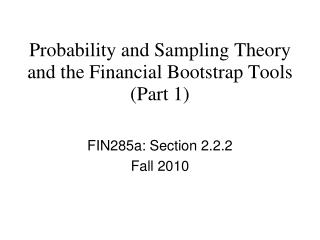DownloadDownload PresentationProbability and Sampling Theory and the Financial Bootstrap Tools (Part 1)

# Probability and Sampling Theory and the Financial Bootstrap Tools (Part 1)

Download Presentation## Probability and Sampling Theory and the Financial Bootstrap Tools (Part 1)

- - - - - - - - - - - - - - - - - - - - - - - - - - - E N D - - - - - - - - - - - - - - - - - - - - - - - - - - -
##### Presentation Transcript

1. FIN285a: Section 2.2.2 Fall 2010 Probability and Sampling Theoryand the Financial Bootstrap Tools(Part 1)

2. Sampling Outline (1) • Sampling • Coin flips • The birthday problem (a not so obvious problem) • Random variables and probabilities • Rainfall • The portfolio (rainfall) problem

3. Financial Bootstrap Commands • sample • count • proportion • quantile • histogram • multiples

4. Software • finboot • coinflip.m • birthday.m • portfolio1.m • portfolio2.m

5. Sampling • Classical Probability/Statistics • Random variables come from static well defined probability distributions or populations • Observe only samples from these populations • Example • Fair coin: (0 1) (1/2 1/2) populations • Sample = 10 draws from this coin

6. Old Style Probability and Statistics • Try to figure out properties of these samples using math formulas • Advantage: • Precise/Mathematical • Disadvantage • Complicated formulas • For relatively complex problems becomes very difficult

7. Bootstrap (resample) Style Probability and Statistics • Go to the computer (finboot toolbox) • Example • coin = [ 0 ; 1] % heads tails • flips = sample(coin,100) • flips = sample(coin,1000) • nheads = count(flips == 0) • ntails = count(flips == 1);

8. Sampling Outline (1) • Sampling • Coin flips • The birthday problem (a not so obvious problem) • Random variables and probabilities • Rainfall • A first portfolio problem

9. The Coin Flip Example • What is the chance of getting fewer than 40 heads in a 100 flips of a fair (50/50) coin? • Could use probability theory, but we'll use the computer • This is a classic binomial distribution (see Jorion 2.4.5) • The computer is not really necessary for this problem

10. Coin Flip Program in Words • Perform 1000 trials • Each trial • Flip 100 coins • Write down how many heads • Summarize • Analyze the distribution of heads • Specifically: Fraction < 40

11. Now to the Computer • coinflip.m and the matlab editor

12. Sampling Outline (1) • Sampling • Coin flips • The birthday problem (a not so obvious problem) • Random variables and probabilities • Rainfall • A portfolio problem

13. Birthday • If you draw 30 people at random what is the probability that two or more have the same birthday?

14. Birthday in Matlab • Each trial • days = sample(1:365,30); • b = multiples(days); • z(trial) = any(b>1) • proportion (z == 1) • on to code

15. Sampling Outline (1) • Sampling • Coin flips and political polls • The birthday problem (a not so obvious problem) • Random variables and probabilities • Rainfall • A portfolio problem

16. Adding Probabilities:Rainfall Example • dailyrain = [80; 10 ; 5 ] • probs = [0.25; 0.5; 0.25]

17. Sampling • annualrain = sum(sample(dailyrain,365,probs))

18. Portfolio Problem • Distribution of portfolio of size 50 • Return of each stock • [ -0.05; 0.0; 0.10] • Prob(0.25,0.5,0.25) • Portfolio is equally weighted • on to matlab code (portfolio1.m)

19. Portfolio Problem 2 • 1 Stock • Return • [-0.05; 0.05] with probability [0.25; 0.75] • Probabilities of runs of positives • 5 days of positive returns • 4/5 days of positive returns • on to matlab code • portfolio2.m

20. Sampling Outline (1) • Sampling • Coin flips • The birthday problem (a not so obvious problem) • Random variables and probabilities • Rainfall • The portfolio (rainfall) problem